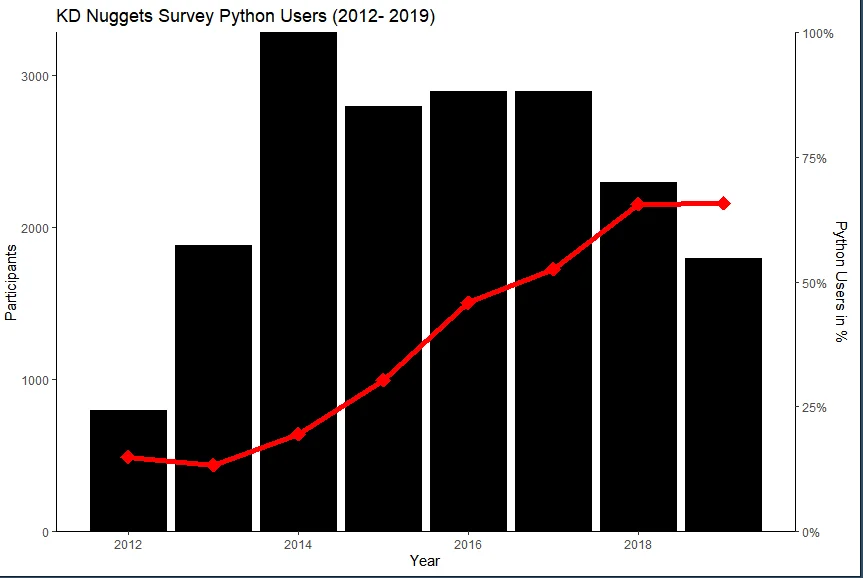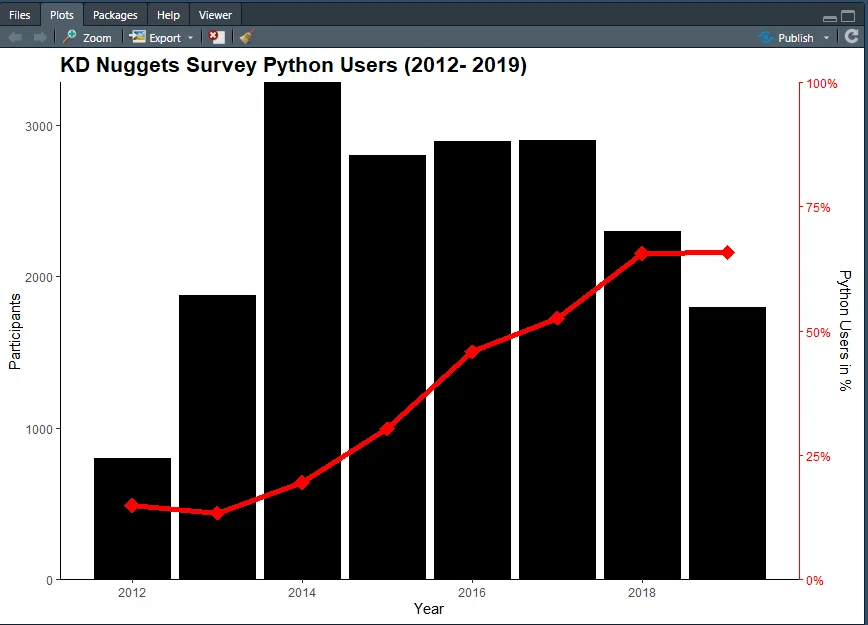25 Jul 2022

Posted on:

16 Jul 2022

0

# Changing the Color of Secondary Axis

Hello, I am trying to make the chart in R as similar as possible to the one in the Python video. To change the color of the secondary axis I used the theme layer: axis.text.y.right, axis.line.y.right, axis.ticks.y.right. But nothing changes on my graph. Any suggestions?

``````library(ggplot2)

sep = ",")

combo <- ggplot(data= df_kdnuggets,
aes(x = Year,
y = Participants, Python.Users)) +
geom_bar( aes ( y = df_kdnuggets\$Participants),
stat = "identity",
fill = "black",
color = "black") +
geom_line(aes( y = df_kdnuggets\$Python.Users*max(df_kdnuggets\$Participants)),
stat = "identity",
color = "red",
size = 2) +
geom_point(aes( y = df_kdnuggets\$Python.Users*max(df_kdnuggets\$Participants)),
color = "red",
size = 5,
shape = 18)+ # change shape to diamond
scale_y_continuous(sec.axis = sec_axis(~./max(df_kdnuggets\$Participants),
labels = scales::percent,
name = "Python Users in %"),
expand = c(0,0)) +
ggtitle("KD Nuggets Survey Python Users (2012- 2019)") +
theme(plot.title = element_text(face = "bold",
color = "black",
size = 16),
axis.text.y.right = element_text(color = "red"),    # Change text to red
axis.line.y.right = element_line(color = "red"),    # change secondary axis to red
axis.ticks.y.right = element_line(color = "red"))+  # change ticks to red

theme_classic()

combo
``````Instructor
Posted on:

25 Jul 2022

0

Hi Diego,
thanks for reaching out! First of all, the chart you've shown looks amazing, congrats on your progress!
I am getting some kind of error when trying out your code, so I'll need a bit more time to troubleshoot the issue. From what I can see, I'd suggest you try and swap the theme_classic and axis.lenght/type/ticks, so that the theme_classic element comes before the axis adjustments. Might be what is causing the issue. Let me know if there's still an issue with the chart.

Best,
365 Eli

Posted on:

25 Jul 2022

0

Hi Eli,

Thank you so much for your help! It works after switching the order of the theme_classic()

``````library(ggplot2)

sep = ",")

combo <- ggplot(data= df_kdnuggets,
aes(x = Year,
y = Participants, Python.Users)) +
geom_bar( aes ( y = df_kdnuggets\$Participants),
stat = "identity",
fill = "black",
color = "black") +
geom_line(aes( y = df_kdnuggets\$Python.Users*max(df_kdnuggets\$Participants)),
stat = "identity",
color = "red",
size = 2) +
geom_point(aes( y = df_kdnuggets\$Python.Users*max(df_kdnuggets\$Participants)),
color = "red",
size = 5,
shape = 18)+ # change shape to diamond
scale_y_continuous(sec.axis = sec_axis(~./max(df_kdnuggets\$Participants),
labels = scales::percent,
name = "Python Users in %"),
expand = c(0,0)) +
ggtitle("KD Nuggets Survey Python Users (2012- 2019)") +
theme_classic()+
theme(plot.title = element_text(face = "bold",
color = "black",
size = 16),
axis.text.y.right = element_text(color = "red"),   # Change text to red
#change secondary axis to red
axis.line.y.right = element_line(color = "red"),
axis.ticks.y.right = element_line(color = "red")) # change ticks to red

combo
``````